# Highlights

• Novel automatic quality evaluation module inspired by the relationship between labels and inputs
• Overfitting control module to ensure enough trainable samples and avoid overfitting
• Method can retain both high accuracy and good generalization at different noise levels

# Introduction

The motivation behind the paper is that it is still a struggle to get a massive amount of clean and precise labels to train a good model, and that low-accuracy segmentation model trained on noisy labels will severely impact the result. The authors also claim that up until now, most approaches for low-quality annotations are designed for the classification tasks.

# Methods

The overall proposed end-to-end architecure is detailed in Figure 2. The authors used the dice loss as segmentation loss and U-Net as their segmentation network.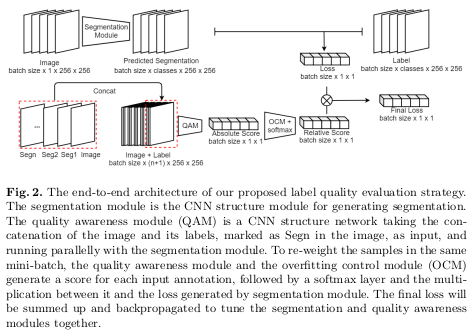## Quality Awareness Module (QAM)

The QAM runs parallel with the segmentation module to calculate weight values to apply to the loss from each sample. During training, the re-weighted loss is used for both the segmentation module and the QAM.

The network used for the QAM is a VGG network, where the number of input channels is changed to $$n + 1$$, where $$n$$ is the number of segmentation classes, to take both image and labels as input. The final layer is also replaced by a one-channel average pooling layer to assess the weight for each sample in the mini-batch.

The resulting loss used by the segmentation model then becomes: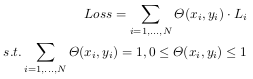The authors mention that they apply a softmax (which does not seem to be pictured in the equation) to rescale all the weights between 0 and 1.

## Overfitting Control Module (OCM)

The motivation behind the OCM is that overfitting problems can arise from the use of the QAM, if the QAM has a larger weight on a small subset with clear-labeled samples. The authors also state that if the QAM makes mistakes during the training process, the relative weight of this sample can be too small or too big for the network to correct.

To counter this, the authors add an extra restriction between the quality awareness module and the softmax layer. They simply use a weighted hyperbolic tangent (as shown in the equation below), to limit the relative ratio of the output quality score from ($$-\infty$$, $$\infty$$) to ($$-\lambda$$, $$\lambda$$). The authors state they empirically set $$\lambda$$ to be 2.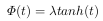## Data

The authors tested their method on the public JSRT dataset, which contains 247 X-rays images of the chest. The labeled structures are the heart, clavicles, and lungs.

To generate noisy-labeled images, the authors used different types and levels of noises. They randomly selected 0%, 25%, 50% and 75% samples from the training set and eroded or dilated them with $$1 \leq n_i \leq 8$$ and $$5 \leq n_i \leq 13$$pixels. Examples of the resulting noisy-labeled segmentations are shown in Figure 1.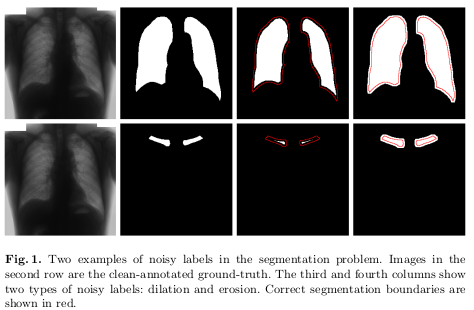# Results

Table 1 and Figure 3 compare the performance of the baseline method and their proposed architecture when faced with more or less noisy labels, and with varying degrees of noise.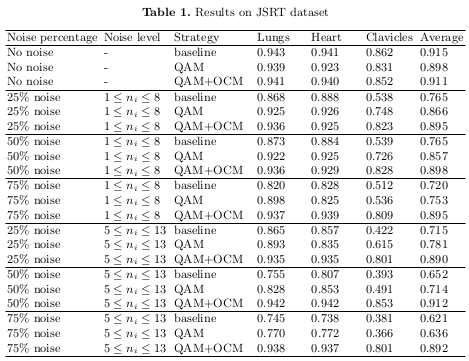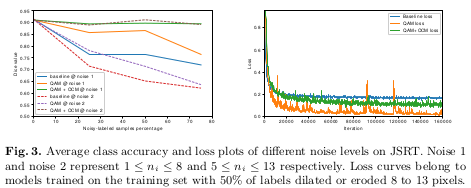Figure 4 gives some insight into how the QAM affects the final loss during the training.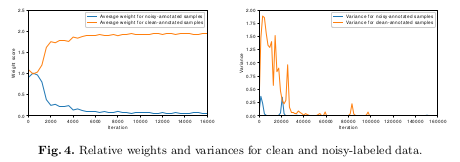# Remarks

• The unclear wording in the paper, along with the lack of details in the network architecture figure, make it unclear whether the QAM uses the original segmentation loss or the re-weighted loss
• No clear explanation (e.g. ablation study) as to why they apply both a softmax and a tanh to the output of the QAM, and whether only one of them would have the same effect (which intuitively would seem to be the case)